# Metric SystemPage 3

#### WATCH ALL SLIDES

density

volume

Slide 19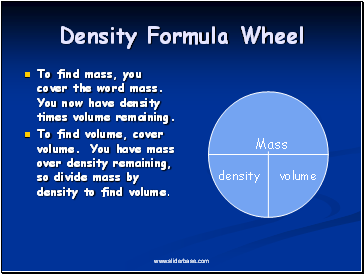## Density Formula Wheel

To find mass, you cover the word mass. You now have density times volume remaining.

To find volume, cover volume. You have mass over density remaining, so divide mass by density to find volume.

Mass

density

volume

Slide 20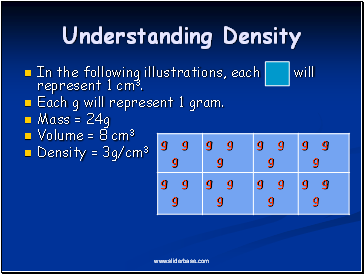## Understanding Density

In the following illustrations, each will represent 1 cm3.

Each g will represent 1 gram.

Mass = 24g

Volume = 8 cm3

Density = 3g/cm3

Slide 21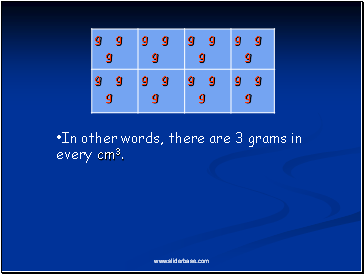In other words, there are 3 grams in every cm3.

Slide 22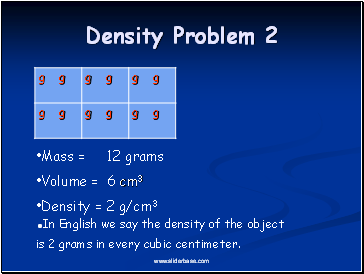Density Problem 2

Mass = 12 grams

Volume = 6 cm3

Density = 2 g/cm3

In English we say the density of the object is 2 grams in every cubic centimeter.

Slide 23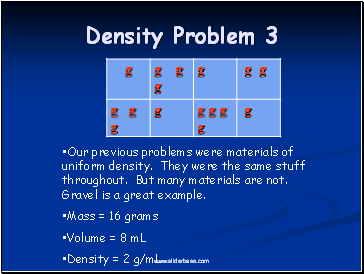Density Problem 3

Our previous problems were materials of uniform density. They were the same stuff throughout. But many materials are not. Gravel is a great example.

Mass = 16 grams

Volume = 8 mL

Density = 2 g/mL

Slide 24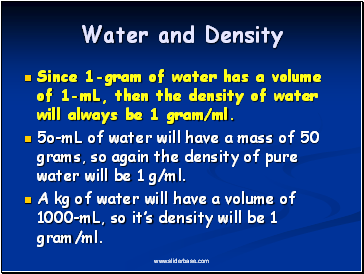## Water and Density

Since 1-gram of water has a volume of 1-mL, then the density of water will always be 1 gram/ml.

5o-mL of water will have a mass of 50 grams, so again the density of pure water will be 1 g/ml.

A kg of water will have a volume of 1000-mL, so it’s density will be 1 gram/ml.

Slide 25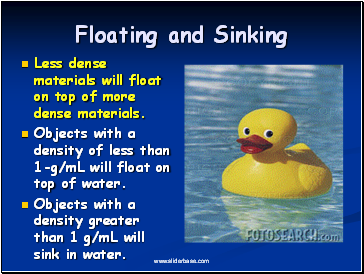## Floating and Sinking

Less dense materials will float on top of more dense materials.

Objects with a density of less than 1-g/mL will float on top of water.

Objects with a density greater than 1 g/mL will sink in water.

Slide 26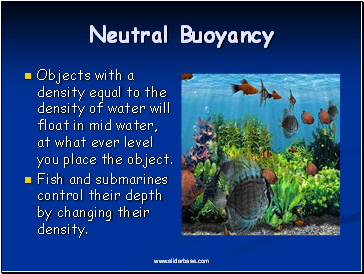## Neutral Buoyancy

Objects with a density equal to the density of water will float in mid water, at what ever level you place the object.

Fish and submarines control their depth by changing their density.

Slide 27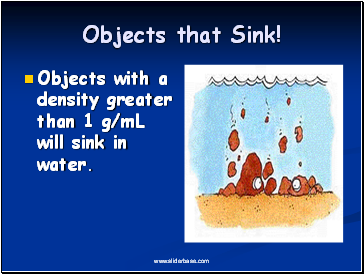## Objects that Sink!

Objects with a density greater than 1 g/mL will sink in water.

Slide 28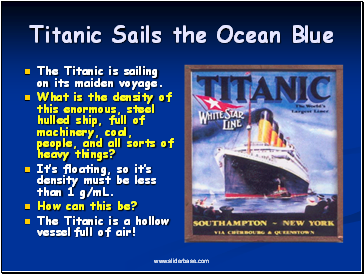## Titanic Sails the Ocean Blue

The Titanic is sailing on its maiden voyage.

What is the density of this enormous, steel hulled ship, full of machinery, coal, people, and all sorts of heavy things?

Go to page:
1  2  3  4